# BATCH.pptx

May. 28, 2023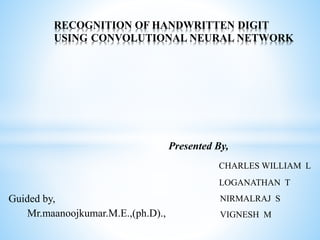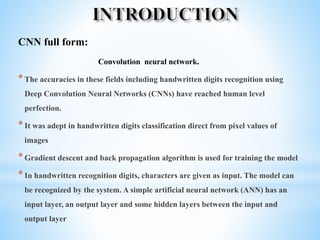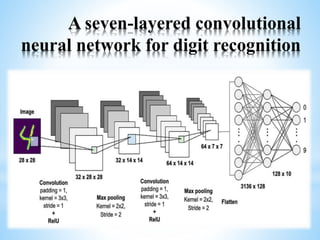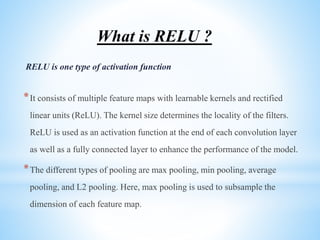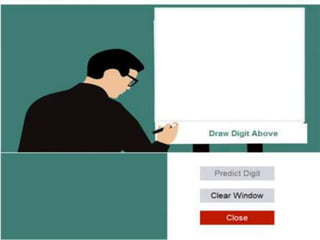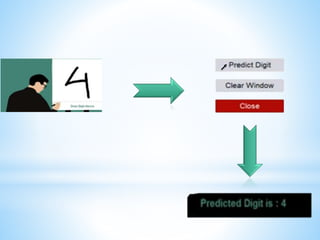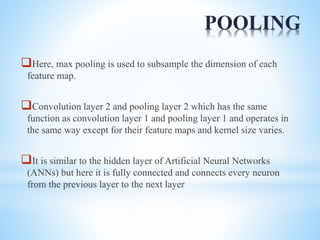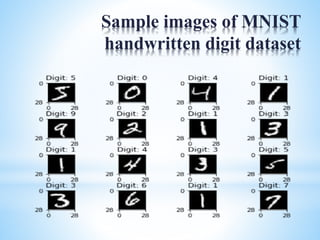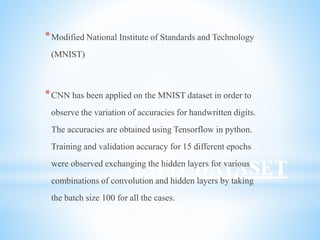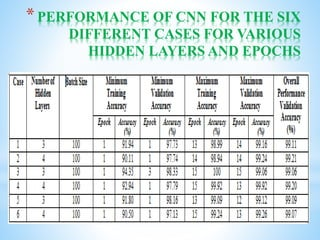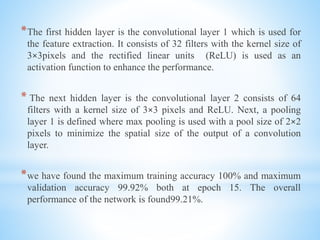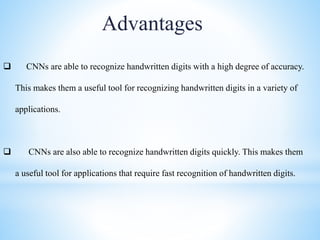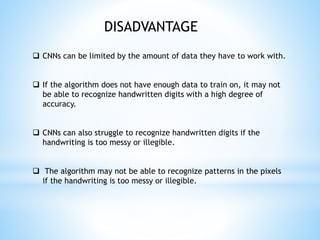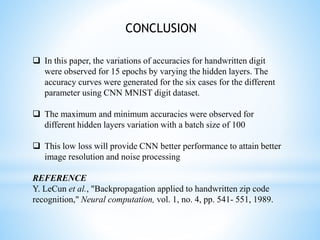1 of 15

### BATCH.pptx

• 1. RECOGNITION OF HANDWRITTEN DIGIT USING CONVOLUTIONAL NEURAL NETWORK Presented By, CHARLES WILLIAM L LOGANATHAN T NIRMALRAJ S VIGNESH M Guided by, Mr.maanoojkumar.M.E.,(ph.D).,
• 2. CNN full form: Convolution neural network. *The accuracies in these fields including handwritten digits recognition using Deep Convolution Neural Networks (CNNs) have reached human level perfection. *It was adept in handwritten digits classification direct from pixel values of images *Gradient descent and back propagation algorithm is used for training the model *In handwritten recognition digits, characters are given as input. The model can be recognized by the system. A simple artificial neural network (ANN) has an input layer, an output layer and some hidden layers between the input and output layer
• 3. A seven-layered convolutional neural network for digit recognition
• 4. What is RELU ? RELU is one type of activation function *It consists of multiple feature maps with learnable kernels and rectified linear units (ReLU). The kernel size determines the locality of the filters. ReLU is used as an activation function at the end of each convolution layer as well as a fully connected layer to enhance the performance of the model. *The different types of pooling are max pooling, min pooling, average pooling, and L2 pooling. Here, max pooling is used to subsample the dimension of each feature map.
• 7. POOLING Here, max pooling is used to subsample the dimension of each feature map. Convolution layer 2 and pooling layer 2 which has the same function as convolution layer 1 and pooling layer 1 and operates in the same way except for their feature maps and kernel size varies. It is similar to the hidden layer of Artificial Neural Networks (ANNs) but here it is fully connected and connects every neuron from the previous layer to the next layer
• 8. Sample images of MNIST handwritten digit dataset
• 9. MNIST DATASET *Modified National Institute of Standards and Technology (MNIST) *CNN has been applied on the MNIST dataset in order to observe the variation of accuracies for handwritten digits. The accuracies are obtained using Tensorflow in python. Training and validation accuracy for 15 different epochs were observed exchanging the hidden layers for various combinations of convolution and hidden layers by taking the batch size 100 for all the cases.
• 10. *PERFORMANCE OF CNN FOR THE SIX DIFFERENT CASES FOR VARIOUS HIDDEN LAYERS AND EPOCHS
• 11. *The first hidden layer is the convolutional layer 1 which is used for the feature extraction. It consists of 32 filters with the kernel size of 3×3pixels and the rectified linear units (ReLU) is used as an activation function to enhance the performance. * The next hidden layer is the convolutional layer 2 consists of 64 filters with a kernel size of 3×3 pixels and ReLU. Next, a pooling layer 1 is defined where max pooling is used with a pool size of 2×2 pixels to minimize the spatial size of the output of a convolution layer. *we have found the maximum training accuracy 100% and maximum validation accuracy 99.92% both at epoch 15. The overall performance of the network is found99.21%.
• 12. Advantages  CNNs are able to recognize handwritten digits with a high degree of accuracy. This makes them a useful tool for recognizing handwritten digits in a variety of applications.  CNNs are also able to recognize handwritten digits quickly. This makes them a useful tool for applications that require fast recognition of handwritten digits.
• 13.  CNNs can be limited by the amount of data they have to work with.  If the algorithm does not have enough data to train on, it may not be able to recognize handwritten digits with a high degree of accuracy.  CNNs can also struggle to recognize handwritten digits if the handwriting is too messy or illegible.  The algorithm may not be able to recognize patterns in the pixels if the handwriting is too messy or illegible. DISADVANTAGE
• 14.  In this paper, the variations of accuracies for handwritten digit were observed for 15 epochs by varying the hidden layers. The accuracy curves were generated for the six cases for the different parameter using CNN MNIST digit dataset.  The maximum and minimum accuracies were observed for different hidden layers variation with a batch size of 100  This low loss will provide CNN better performance to attain better image resolution and noise processing REFERENCE Y. LeCun et al., "Backpropagation applied to handwritten zip code recognition," Neural computation, vol. 1, no. 4, pp. 541- 551, 1989. CONCLUSION